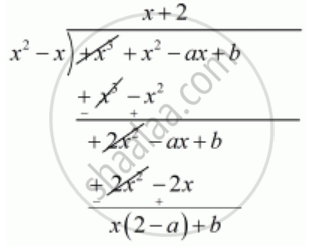Advertisement Remove all ads

# If F(X) = X3 + X2 − Ax + B is Divisible by X2 − X Write the Value of a and B. - Mathematics

Sum

If f(x) = x3 + x2 − ax + b is divisible by x2 − x write the value of a and b.

Advertisement Remove all ads

#### Solution

We are given f(x) = x3 + x2 − ax + b  is exactly divisible by  x2 − x then the remainder should be zeroTherefore Quotient  = x+2 and

Remainder = x(2 -a)+ b

Now, Remainder  = 0

x (2 - a)+ b = 0

x ( 2 - a) + b = 0 x + 0

Equating coefficient of x, we get

2 - a = 0

 2 = a

Equating constant term

b = 0

Hence, the value of a and b are   a= 2 , b = 0

Is there an error in this question or solution?
Advertisement Remove all ads

#### APPEARS IN

RD Sharma Class 10 Maths
Chapter 2 Polynomials
Q 24 | Page 60
Advertisement Remove all ads

#### Video TutorialsVIEW ALL 

Advertisement Remove all ads
Share
Notifications

View all notifications

Forgot password?